GeeksforGeeks App
Open AppBrowser
Continue

# Differential or Derivatives in MATLAB

Differentiation of a function y = f(x) tells us how the value of y changes with respect to change in x. It can also be termed as the slope of a function.

Derivative of a function f(x) wrt to x is represented asMATLAB allows users to calculate the derivative of a function using diff() method. Different syntax of diff() method are:

• f’ = diff(f)
• f’ = diff(f, a)
• f’ = diff(f, b, 2)

### f’ = diff(f)

It returns the derivative of function f(x) wrt variable x.

Example 1:

## Matlab

 % Create a symbolic expression in variable xsyms xf = cos(x);disp("f(x) :");disp(f); % Derivative of f(x)d = diff(f);disp("Derivative of f(x) :");disp(d);

Output :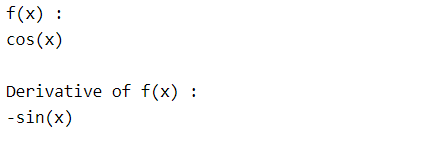Example 2: Evaluating the derivative of a function at a specified value using subs(y,x,k).

• subs(y,x,k), it gives the value of function y at x = k.

## Matlab

 % Create a symbolic expression in# variable xsyms xf = cos(x);disp("f(x) :");disp(f); % Derivative of f(x)d = diff(f);val = subs(d,x,pi/2); disp("Value of f'(x) at x = pi/2:");disp(val);

Output :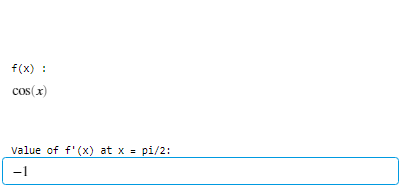### f’ = diff(f, a)

• It returns the derivative of function f with respect to variable a.

## Matlab

 % Create a symbolic expression in variable xsyms x t;f = sin(x*t);disp("f(x) :");disp(f); % Derivative of f(x,t) wrt td = diff(f,t);disp("Derivative of f(x,t) wrt t:");disp(d);

Output :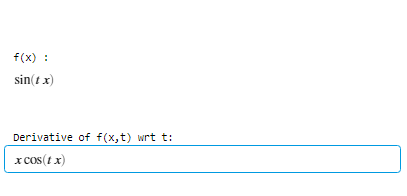### f’ = diff(f, b, 2)

It returns the double derivative of function f with respect to variable b.

Example 1:

## Matlab

 % Create a symbolic expression in% variable x,nsyms x n;f = x^n;disp("f(x,n) :");disp(f); % Double Derivative of f(x,n) wrt xd = diff(f,x,2);disp("Double Derivative of f(x,n) wrt x:");disp(d);

Output :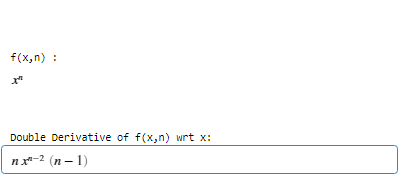In the same way, you can also calculate the k-order derivative of function f using diff(f,x,k).

Example 2:

Calculating the partial derivative} using Jacobian matrix and determinant.

•## Matlab

 % Create a symbolic expression in variable% u and vsyms u v;f = u^2;g = sin(v)*(3*u);disp("f(u,v) :");disp(f);disp("g(u,v) :");disp(g); % Jacobian matrix of function f(u,v) and% g(u,v)J = jacobian([f; g], [u v]);disp("Jacobian matrix :");disp(J); % Determinant of Jacobian matrixd = det(J);disp("Determinant of Jacobian matrix:");disp(d);

Output :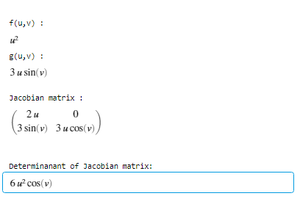My Personal Notes arrow_drop_up